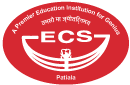## Maths Lab

A child can perceive a concept thoroughly only with the combination of theoretical teaching in a classroom and hands on experience in a lab. Math needs practice, interest and brainpower which are fostered by the Math lab. The Math Lab makes Math simple and fun for students. The lab is well equipped to demonstrate and elucidate any topic.

For instance, the ‘Ramanujan’s Kit’ has all the components with which every important concept and formula can be proved practically. It enables the teachers to demonstrate and reinforce Mathematical concepts. Hence, it helps in mental enhancement in areas of Math like Algebra, Arithmetic, Calculus, Geometry, Statistics, and Trigonometry etc.